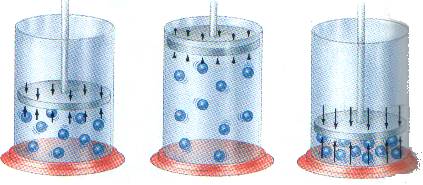# Boyle's LawBOYLE'S LAW

• OBJECTIVES

• Describe in qualitative terms the effects of changes in pressure and volume on contained gases.

• Calculate pressure or volume from the pressure - volume relationship of a contained gas at constant temperature.

• PROBLEM

• Variation between the pressure and volume.

• HYPOTHESIS

• The pressure and volume vary inversely proportional.

• MATERIALS

• A syringe

• A graphic calculator

• CBL

• A pressure sensor.

• PROCEDURE

• Turn on CBL and the graphic calculator.

• To program Chembio.

• To put prove, channel, calibration, used stored in that order.

• To press Triger in CBL after each measure bye the pressure sensor.

• When you finish all measures to put in the graphic calculator “No repeat”, first curve, and Power L1, L2.

• DATA

• The equation for calculate the heat released is:

•  P1V1 = P2V2
• P = pressure

• V = volume

• OBSERVATIONS

• Table Nº1

 Volume (L) Pressure (Kpa) 2.5 179.87 5.0 101.18 7.5 72.45 10.0 56.21 12.5 46.22 15.0 38.72 17.5 33.73
• ANALYSIS

• CONCLUSIONS

• When the pressure goes up, the volume goes down.

• The relationship between pressure and volume is inversely proportional.

• The product of volume and pressure at any two sets of conditions is always constant at a given temperature.

• Pressure increased when volume decreased because the gas molecules have minor space and the container received most fight by the molecules inside its.

• The collision of the particles in a gas with the walls of the container is gas pressure.

• The pressure in the container increases in proportion to the number of gas particles inside its.

• The gases' constituent corpuscles stand still always they are in contact whit others.

• The gases' constituent corpuscles can compress them, this one explains their capacity for to decreased the volume when a exterior pressure affects them.

• The volume of a container easily accommodates whichever number of particles.

• The mechanics process in a laboratory make, sometimes, we obtain wrong answers.

•Enviado por: El remitente no desea revelar su nombre Idioma: inglés País: Estados Unidos

Palabras clave:
Te va a interesar